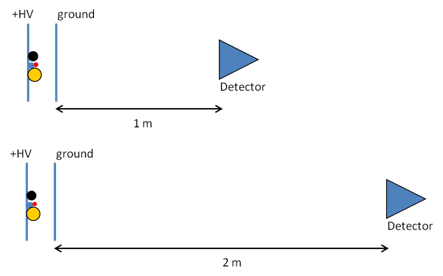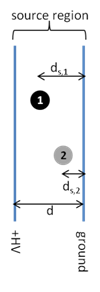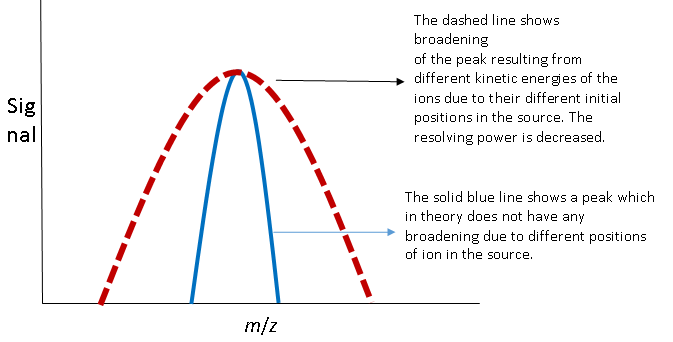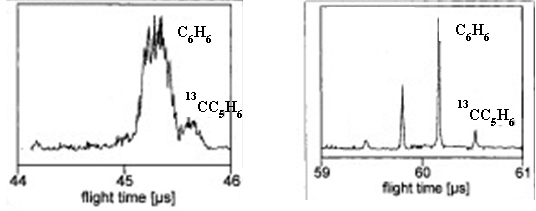# Section 3B. Time of Flight (TOF) Mass Analyzer

$$\newcommand{\vecs}{\overset { \rightharpoonup} {\mathbf{#1}} }$$ $$\newcommand{\vecd}{\overset{-\!-\!\rightharpoonup}{\vphantom{a}\smash {#1}}}$$$$\newcommand{\id}{\mathrm{id}}$$ $$\newcommand{\Span}{\mathrm{span}}$$ $$\newcommand{\kernel}{\mathrm{null}\,}$$ $$\newcommand{\range}{\mathrm{range}\,}$$ $$\newcommand{\RealPart}{\mathrm{Re}}$$ $$\newcommand{\ImaginaryPart}{\mathrm{Im}}$$ $$\newcommand{\Argument}{\mathrm{Arg}}$$ $$\newcommand{\norm}{\| #1 \|}$$ $$\newcommand{\inner}{\langle #1, #2 \rangle}$$ $$\newcommand{\Span}{\mathrm{span}}$$ $$\newcommand{\id}{\mathrm{id}}$$ $$\newcommand{\Span}{\mathrm{span}}$$ $$\newcommand{\kernel}{\mathrm{null}\,}$$ $$\newcommand{\range}{\mathrm{range}\,}$$ $$\newcommand{\RealPart}{\mathrm{Re}}$$ $$\newcommand{\ImaginaryPart}{\mathrm{Im}}$$ $$\newcommand{\Argument}{\mathrm{Arg}}$$ $$\newcommand{\norm}{\| #1 \|}$$ $$\newcommand{\inner}{\langle #1, #2 \rangle}$$ $$\newcommand{\Span}{\mathrm{span}}$$

This set of questions guides students through the principle of a TOF mass analyzer. There is very little reading and all questions are for small group discussion.

(Source for figures and animations: Dr. Jon Karty of Indiana University Mass Spectrometry Facility)Consider the diagram above, which shows 4 ions (the circles), between two electrical plates. The ions have different masses and higher mass is represented by a larger circle. Imagine the entire schematic is contained in a long tube under vacuum. After the ions enter, the left plate is brought to a positive high voltage (+HV) such as +15,000 V. The right plate is a grid, like a screen door, so that ions can pass through, and is held at electrical ground (0 V).

1. A. They will move away from the plate because like charges repel.
2. A. The smallest ion (red) with the lowest mass will move fastest and reach the detector first.

The powerpoint file, TOF Animations Slide 2 has a nice animation showing the answer to this question.

3. A. The magnitude of the positive voltage and the magnitude of the charge on the ion. Also, the distance the ion travels through the electric field.

Note: Most students will not know about the effect of distance through the electric field. This phenomena limits resolution and with be developed later on this worksheet.

4. This time of flight mass analyzer uses an applied voltage (+HV) to give kinetic energy (KE) to the ions. The amount of energy imparted to the ions is given by Equation $$\ref{1}$$:

$KE = zV \label{1}$

where z is the charge on the ion and V is the magnitude of the high voltage (HV).

A. Yes (The charge on the ions and the applied voltage influence kinetic energy.)

2. If all of the ions have the same charge, what will be true about their kinetic energies?

A. They will all have the same kinetic energy (KE) because they are all accelerated with the same voltage.

5. You may remember another equation for kinetic energy from physics:

$KE = \dfrac{1}{2} mv^2 \label{2}$

where m is the mass of the object and v is its velocity.

1. Consider the four ions at the start of this worksheet. Which ion will have the highest velocity?

A. If ions with the same charge have the same kinetic energy, then lighter ions will have a higher velocity. The red ion has the smallest mass and will have the highest velocity.

2. What is the order of ions reaching the detector?

A. The order of ions reaching the detector is lowest to highest mass. Red, blue, black, yellow. ‘

3. Is the TOF mass analyzer dispersive or scanning?

A. Dispersive because changes do not need to be made to electric or magnetic fields to pass an ion with a given m/z to the detector. The flight times are very short (on the order of µs and therefore ions are essentially detected all at once allowing for collection of many signals in a short amount of time. The laser can pulse 200 times per second allowing 200 signals to be averaged in 1 second greatly improving signal to noise ratio. Note to instructors: This question could be omitted if you have not previously discussed dispersive vs. scanning mass analyzers in the course.

4. Why do you think it is called a “time-of-flight” mass analyzer?

A. Ions of different m/z are separated because they take different amounts of time to reach the detector.

5. Consider a +1 ion with m/z = 115 which enters the time-of-flight source region (between the two plates).

If +HV = 2000 V, how much kinetic energy is imparted to the ion in Joules?

1 elementary charge = 1.6 × 10-19 C $$\mathrm{1\: V = 1\:\: \large{ {}^J/_C} }$$

A. KE = zV = (+1)(1.6 × 10-19 C)(2000 V) = 3.2 × 10-16 C . V = 3.2 × 10-16 J

6. What is the velocity of the ion in m/s?

$$\mathrm{1\: J = 1\: \dfrac{kg \cdot m^2}{s^2}}$$ 1 amu = 1.66 × 10-27 kg

$$KE = \left(\dfrac{1}{2} \right ) mv^2$$

$$3.2\times10^{-16}\dfrac{kg\cdot m^2}{s^2} = \left(\dfrac{1}{2} \right )(115\: amu)\left(\dfrac{1.66\times10^{-27}\:kg}{amu} \right )v^2$$

$$v = \mathrm{5.8\times 10^4\: m/s}$$

7. If the flight tube is 2 m long, how long will the ion take to reach the detector? Remember that v = d/t, where v is velocity, d is distance traveled, and t is time.

A. 3.5 × 10-5 s or 35 μs

6. The ability of a mass spectrometer to distinguish between two different m/z ions is called resolving power.

A common definition for resolving power is the m/z of the peak (m) divided by the width of the peak at half maximum height (Δm).

$$\textrm{Resolving Power} = \dfrac{m}{\Delta m}$$Image adapted from UC Davis Fiehn Metabolomics Lab. fiehnlab.ucdavis.edu/projects...ass_Resolution

1. Calculate the resolving power of the mass analyzer from the peak in the figure.

A. $$\textrm{Resolving Power} = \dfrac{m}{\Delta m} = \dfrac{500.00}{0.10} = 5000 = 5.0 \times 10^3$$

2. Using the resolving power calculated in part (a), sketch the appearance of the two peaks with m/z ratios of 1500 and 1500.5. Would you say these peaks are resolved?

A. The figure shows the appearance of the two peaks. They are resolved because they do not overlap at the baseline.7. Consider the two instrument schematics for a time of flight mass analyzer below. Which one do you think will be better at resolving small differences in the masses of the ions? Explain.A. The longer flight tube will result in better resolution because the ions with different speeds will have more time to separate. Imagine two runners. One runner has a pace of 7:00 per mile, the other runner has a pace of 8:00 per mile. After 100 yards there will not be much separation between the two, but after a mile the separation will be much larger.

8. Consider the possibility diagrammed below, in which two ions of the same mass and charge start at slightly different positions in the source region.1. Which ion will leave the source region with the higher velocity, Ion 1 or Ion 2?

A. Ion 1

Equation $$\ref{1}$$ can also be written as:

$KE=zEd_s \tag{3.1b}$

where E is the electric field (V/d) and ds is the distance traveled in the source region. Use this equation to explain which ion (1 or 2) will leave the source with the highest velocity.

A. Ion 1 will be accelerated a larger distance through the electric field thus acquiring greater kinetic energy and velocity. The powerpoint file, TOF Animations, Slide 9 has a nice animation showing how the position of the ion in source affects the flight time.

2. How will the position of different ions in the source affect the resolving power of the mass analyzer? Draw a peak for a given m/z similar to the one in question 7 assuming that the position of the ion in the source did not affect kinetic energy. Draw a new peak taking into account how different positions of ions in the source affect the kinetic energy of the ions.

A. The resolving power will decrease because ions of the same m/z formed at different locations in the source will acquire different kinetic energies and reach the detector at different times. In theory, ions with the same m/z should have the same kinetic energy and reach the detector at the same time. The effect of different positions of ions in the source will broaden the peak.9. Consider the instrument diagram below, which adds a “reflectron” to the mass analyzer. The reflectron consists of a series of grids held at increasingly high positive potentials from ground (0 V) up to +HV2. Note: +HV2 > +HV1.1. On the diagram above, sketch the flight paths for Ion 1 and Ion 2 (these ions have the same m/z ratio and are the same ions in question 8). Note that the reflectron is angled toward the detector.

A. Ion 1 and 2 turn around at the reflection and head toward the detector.

2. Which ion penetrates farther into the reflectron?

A. Ion 1 will leave the source with greater kinetic energy and will be more difficult to stop and turn around. Therefore Ion 1 will penetrate further into the reflectron.

3. How does this arrangement correct for the ions’ different starting positions in the source?

A. Ion 1 is moving faster but travels a greater distance because it penetrates further into the reflectron. Ion 1 and Ion 2 should reach the detector at nearly the same time.

The Powerpoint file, TOF Animations Slide 10 shows the effect of the reflectron on the flight time of two ions with the same m/z formed at different positions in the source.

10. Examine the diagram of a reflectron TOF mass analyzer.1. What is the order of ions reaching the detector in the reflectron time of flight mass spectrometer? (all ions have a +1 charge)

A. Lowest mass ion arrives first and highest mass ion arrives last. (red, blue, black, then yellow)

2. Why is the ionization method MALDI frequently coupled with a time of flight mass analyzer?

A. To measure flight times a set of ions must enter the mass analyzer and be accelerated all at the same time. The pulsed nitrogen laser produces a “pulse” of ions for time of flight analysis. The nitrogen laser is often pulsed 100-200 times per second leading to improved signal to noise ratio due to signal averaging.

3. Examine the two mass spectra below. Identify which spectrum is from a reflectron TOF instrument and which is from a linear TOF instrument?A.Section 3B. Time of Flight (TOF) Mass Analyzer is shared under a not declared license and was authored, remixed, and/or curated by LibreTexts.